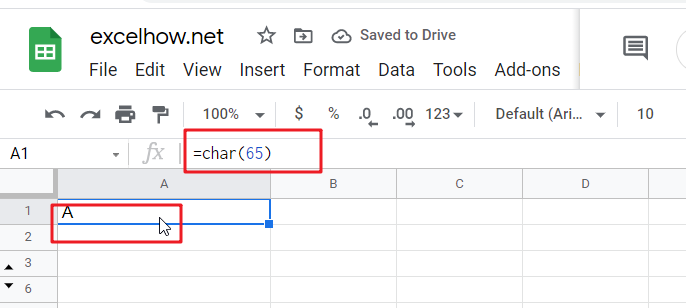This post will guide you how to use Google Sheets CHAR function with syntax and examples.

## 1. Google Sheets CHAR Function Description

The Google Sheets CHAR function returns the character specified by a number (ASCII Value) according to the current Unicode table. And you can get the Unicode table online at the Unicode website.

The CHAR function can be used to specify a character that are hard to enter in a formula in google sheets. For example, if you wish to enter a line break, and you can use the CHAR(10) function to add a line break to  a text string in a formula in google sheets.

The CHAR function is a build-in function in Google Sheets and it is categorized as a Text Function.

## 2. Google Sheets CHAR Function Syntax

The syntax of the CHAR function is as below:

``=CHAR(number)``

Where the CHAR function argument is:

Number -This is a required argument. the number value is in decimal format and the number of character to look up from the current Unicode table in decimal format.

Note:

• If you provided invalided number, such as: a string value, you will get an error from the Google Sheets CHAR function, it is like to be the “#VALUE!” error
• The CHAR function only have one argument, which must be an valid number in decimal format. The result from Google Sheets CHAR function is a text value. And the CHAR function can be useful when you want to specify a character in formulas or function that are hard to type directly.
• You need to know that not all Unicode characters can be display properly on your computers and you may need to install or enable special fonts or languages so that it can be displayed properly.

## 3. Google Sheets CHAR Function Example

#1 get a character from number 65, 33,  in the computer’s character set

#2 enter one Double Quotes with text in a formula

If you wish to concatenate double quotes with text in Google sheets, and you can use the CHAR function with a number 34 as its argument, type:

=CHAR(34)

## 4. Conclusion

You need know that the CHAR function will only return a single character. If you want to return a string of characters, you can use the CONCATENATE function to combine multiple CHAR functions.

This post will guide you how to use Google Sheets CODE  function with syntax and examples.

## Description

The Google Sheets CODE function returns the numeric Unicode map value for the first character of a text string. And you can get the Unicode table online at the Unicode website.

The CODE function can be used to get a numeric code for a given character or a text string. For example, if you wish to get a Unicode map value of the character “a”, and you can use the CODE(‘a’) function to get the numeric code 97 in a formula in google sheets.

The CODE function is a build-in function in Google Sheets and it is categorized as a Text Function.

## Syntax

The syntax of the CODE function is as below:

=CODE(text)

Where the CODE function argument is:

Text -This is a required argument. The text strings that you want to get the numeric Unicode map value of the first character

Note:

• You need to know that the CODE function will only convert the first character in a text string and other characters will be ignored.
• The CODE function only takes one argument, which is normally a text string or character. If the text value contains more than one character, and the CODE function only returns a Unicode map value for the first character.
• The CODE function only handle numeric input for the numbers 0-9.

## Example

#1 To get the numeric Unicode map value of the character` “c”`, just using the following CODE function:

=CODE(“c”)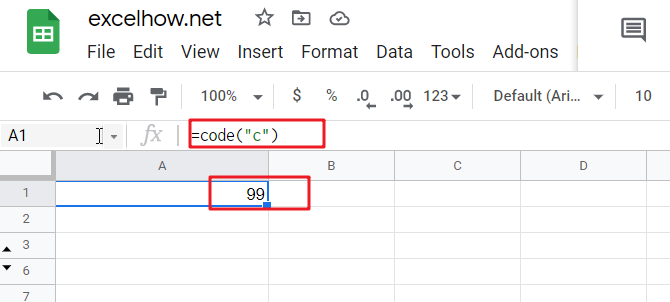#2 To get the numeric Unicode map value of the character `“c”` in cell A1, just using the following CODE function:

=CODE(A1)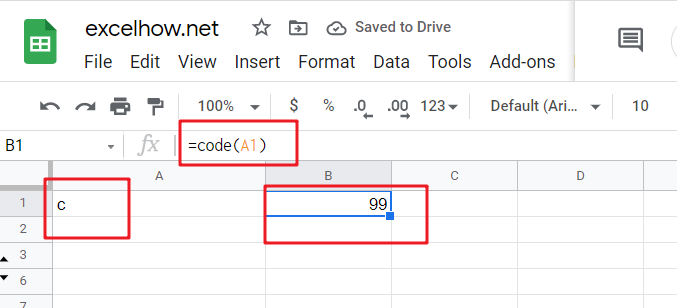#3 To get the numeric Unicode map value of string `“code”`, just using the following CODE function:

=CODE(“code”)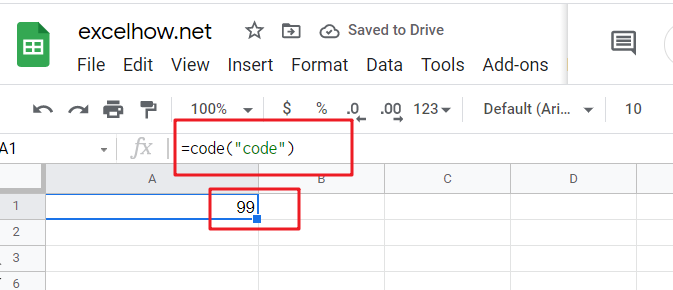#4 To get the numeric Unicode map value of numeric input for number `1`, using the following CODE function:

=CODE(1)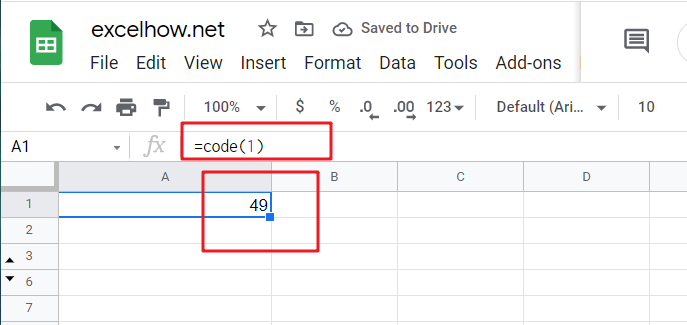So the numeric value returned by the CODE function is the code for a character in Unicode table.

If you want to get a character for given numeric code in Unicode table, and you can use the CHAR function, For example, if you want to get a Unicode character for a numeric value `65`, and you can use the following CHAR function:

=CHAR(65)